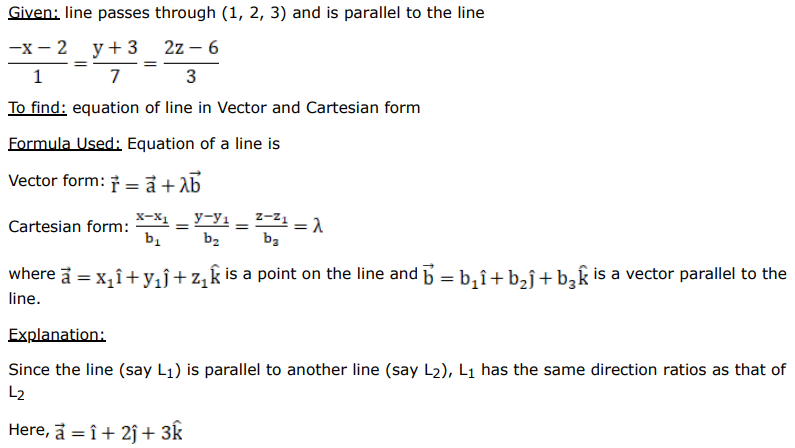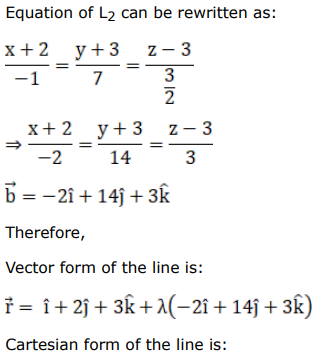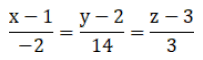# Find the Cartesian and vector equations of a line which passes through the point

Question:

Find the Cartesian and vector equations of a line which passes through the point $(1,2,3)$ and is parallel to the line $\frac{-x-2}{1}=\frac{y+3}{7}=\frac{2 z-6}{3}$.

Solution: